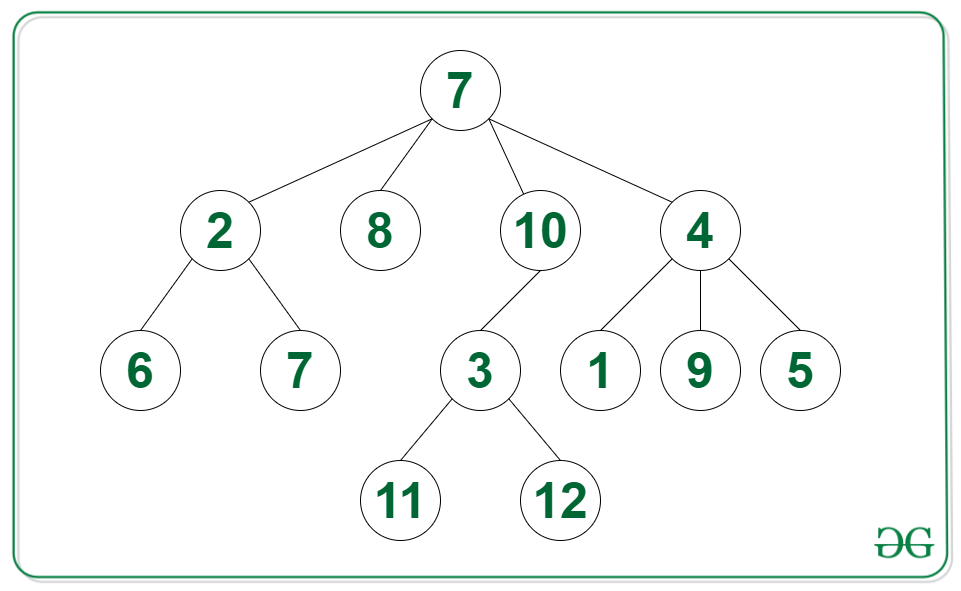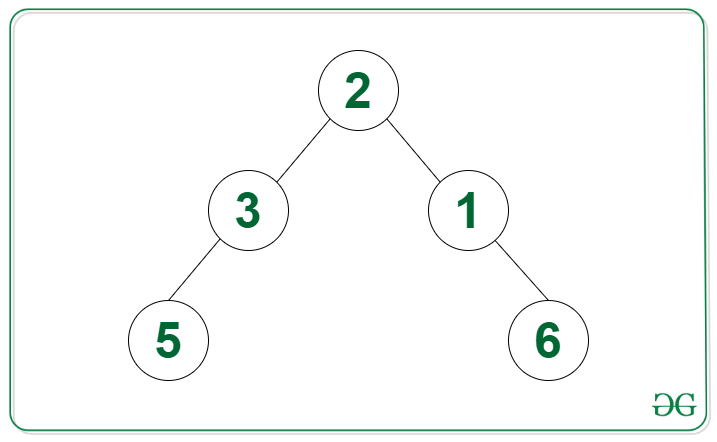Open in App
Not now

# Count of nodes in a given N-ary tree having distance to all leaf nodes equal in their subtree

• Last Updated : 11 Jan, 2022

Given an N-ary tree root, the task is to find the number of non-leaf nodes in the tree such that all the leaf nodes in the subtree of the current node are at an equal distance from the current node.

Example:

Input: Tree in the below image
Output: 4
Explanation: The nodes {10, 3, 2, 4} have the distance between them and all the leaf nodes in their subtree respectively as equal.Input: Tree in the image below
Output: 3Approach: The given problem can be solved by using the post-order traversal. The idea is to check if the number of nodes from the current node to all its leaf nodes is the same. Below steps can be followed to solve the problem:

• Apply post-order traversal on the N-ary tree:
• If the root has no children then return 1 to the parent
• If every branch has equal height then increment the count by 1 and return height + 1 to the parent
• Or else return -1 to the parent indicating that the branches have unequal height
• Return the count as the answer

## C++

 `// C++ code for the above approach``#include ``using` `namespace` `std;``class` `Node {``public``:``    ``vector children;``    ``int` `val;``    ``// constructor``    ``Node(``int` `v)``    ``{``        ``val = v;``        ``children = {};``    ``}``};` `// Post-order traversal to find``// depth of all branches of every``// node of the tree``int` `postOrder(Node* root, ``int` `count[])``{``  ` `    ``// If root is a leaf node``    ``// then return 1``    ``if` `(root->children.size() == 0)``        ``return` `1;``  ` `    ``// Initialize a variable height``    ``// calculate longest increasing path``    ``int` `height = 0;``  ` `    ``// Use recursion on all child nodes``    ``for` `(Node* child : root->children)``    ``{``      ` `        ``// Get the height of the branch``        ``int` `h = postOrder(child, count);``      ` `        ``// Initialize height of first``        ``// explored branch``        ``if` `(height == 0)``            ``height = h;``      ` `        ``// If branches are unbalanced``        ``// then store -1 in height``        ``else` `if` `(h == -1 || height != h)``            ``height = -1;``    ``}``  ` `    ``// Increment the value of count``    ``// If height is not -1``    ``if` `(height != -1)``        ``count++;``  ` `    ``// Return the height of branches``    ``// including the root if height is``    ``// not -1 or else return -1``    ``return` `height != -1 ? height + 1 : -1;``}` `// Function to find the number of nodes``// in the N-ary tree with their branches``// having equal height``int` `equalHeightBranches(Node* root)``{``  ` `    ``// Base case``    ``if` `(root == NULL)``        ``return` `0;``  ` `    ``// Initialize a variable count``    ``// to store the answer``    ``int` `count = { 0 };``  ` `    ``// Apply post order traversal``    ``// on the tree``    ``postOrder(root, count);``  ` `    ``// Return the answer``    ``return` `count;``}``// Driver code``int` `main()``{``    ``// Initialize the tree``    ``Node* seven = ``new` `Node(7);``    ``Node* seven2 = ``new` `Node(7);``    ``Node* five = ``new` `Node(5);``    ``Node* four = ``new` `Node(4);``    ``Node* nine = ``new` `Node(9);``    ``Node* one = ``new` `Node(1);``    ``Node* two = ``new` `Node(2);``    ``Node* six = ``new` `Node(6);``    ``Node* eight = ``new` `Node(8);``    ``Node* ten = ``new` `Node(10);``    ``Node* three = ``new` `Node(3);``    ``Node* mfour = ``new` `Node(-4);``    ``Node* mtwo = ``new` `Node(-2);``    ``Node* zero = ``new` `Node(0);``    ``three->children.push_back(mfour);``    ``three->children.push_back(mtwo);``    ``three->children.push_back(zero);``    ``ten->children.push_back(three);``    ``two->children.push_back(six);``    ``two->children.push_back(seven2);``    ``four->children.push_back(nine);``    ``four->children.push_back(one);``    ``four->children.push_back(five);``    ``seven->children.push_back(ten);``    ``seven->children.push_back(two);``    ``seven->children.push_back(eight);``    ``seven->children.push_back(four);``  ` `    ``// Call the function``    ``// and print the result``    ``cout << (equalHeightBranches(seven));``}` `// This code is contributed by Potta Lokesh`

## Java

 `// Java implementation for the above approach` `import` `java.io.*;``import` `java.util.*;` `class` `GFG {` `    ``// Function to find the number of nodes``    ``// in the N-ary tree with their branches``    ``// having equal height``    ``public` `static` `int` `equalHeightBranches(Node root)``    ``{` `        ``// Base case``        ``if` `(root == ``null``)``            ``return` `0``;` `        ``// Initialize a variable count``        ``// to store the answer``        ``int``[] count = ``new` `int``[``1``];` `        ``// Apply post order traversal``        ``// on the tree``        ``postOrder(root, count);` `        ``// Return the answer``        ``return` `count[``0``];``    ``}` `    ``// Post-order traversal to find``    ``// depth of all branches of every``    ``// node of the tree``    ``public` `static` `int` `postOrder(``        ``Node root, ``int``[] count)``    ``{` `        ``// If root is a leaf node``        ``// then return 1``        ``if` `(root.children.size() == ``0``)``            ``return` `1``;` `        ``// Initialize a variable height``        ``// calculate longest increasing path``        ``int` `height = ``0``;` `        ``// Use recursion on all child nodes``        ``for` `(Node child : root.children) {` `            ``// Get the height of the branch``            ``int` `h = postOrder(child, count);` `            ``// Initialize height of first``            ``// explored branch``            ``if` `(height == ``0``)``                ``height = h;` `            ``// If branches are unbalanced``            ``// then store -1 in height``            ``else` `if` `(h == -``1` `|| height != h)``                ``height = -``1``;``        ``}` `        ``// Increment the value of count``        ``// If height is not -1``        ``if` `(height != -``1``)``            ``count[``0``]++;` `        ``// Return the height of branches``        ``// including the root if height is``        ``// not -1 or else return -1``        ``return` `height != -``1` `? height + ``1` `: -``1``;``    ``}` `    ``// Driver code``    ``public` `static` `void` `main(String[] args)``    ``{` `        ``// Initialize the tree``        ``Node seven = ``new` `Node(``7``);``        ``Node seven2 = ``new` `Node(``7``);``        ``Node five = ``new` `Node(``5``);``        ``Node four = ``new` `Node(``4``);``        ``Node nine = ``new` `Node(``9``);``        ``Node one = ``new` `Node(``1``);``        ``Node two = ``new` `Node(``2``);``        ``Node six = ``new` `Node(``6``);``        ``Node eight = ``new` `Node(``8``);``        ``Node ten = ``new` `Node(``10``);``        ``Node three = ``new` `Node(``3``);``        ``Node mfour = ``new` `Node(-``4``);``        ``Node mtwo = ``new` `Node(-``2``);``        ``Node zero = ``new` `Node(``0``);``        ``three.children.add(mfour);``        ``three.children.add(mtwo);``        ``three.children.add(zero);``        ``ten.children.add(three);``        ``two.children.add(six);``        ``two.children.add(seven2);``        ``four.children.add(nine);``        ``four.children.add(one);``        ``four.children.add(five);``        ``seven.children.add(ten);``        ``seven.children.add(two);``        ``seven.children.add(eight);``        ``seven.children.add(four);` `        ``// Call the function``        ``// and print the result``        ``System.out.println(``            ``equalHeightBranches(seven));``    ``}` `    ``static` `class` `Node {` `        ``List children;``        ``int` `val;` `        ``// constructor``        ``public` `Node(``int` `val)``        ``{` `            ``this``.val = val;``            ``children = ``new` `ArrayList<>();``        ``}``    ``}``}`

## Python3

 `# Python code for the above approach``class` `Node:``    ``def` `__init__(``self``, val):``        ``self``.val ``=` `val``        ``self``.children ``=` `[]` `# Post-order traversal to find``# depth of all branches of every``# node of the tree``def` `postOrder(root, count):` `        ``# If root is a leaf node``        ``# then return 1``    ``if` `(``len``(root.children) ``=``=` `0``):``        ``return` `1` `    ``# Initialize a variable height``    ``# calculate longest increasing path``    ``height ``=` `0` `    ``# Use recursion on all child nodes``    ``for` `child ``in` `root.children:` `        ``# Get the height of the branch``        ``h ``=` `postOrder(child, count)` `        ``# Initialize height of first``        ``# explored branch``        ``if` `(height ``=``=` `0``):``            ``height ``=` `h` `        ``# If branches are unbalanced``        ``# then store -1 in height``        ``elif` `(h ``=``=` `-``1` `or` `height !``=` `h):``            ``height ``=` `-``1` `    ``# Increment the value of count``    ``#  If height is not -1``    ``if` `(height !``=` `-``1``):``        ``count[``0``] ``+``=` `1` `    ``# Return the height of branches``    ``# including the root if height is``    ``# not -1 or else return -1``    ``if``(height !``=` `-``1``):``        ``return` `height ``+` `1``    ``else``:``        ``return` `-``1` `# Function to find the number of nodes``# in the N-ary tree with their branches``# having equal height``def` `equalHeightBranches(root):` `    ``# Base case``    ``if` `(root ``=``=` `None``):``        ``return` `0` `    ``# Initialize a variable count``    ``# to store the answer``    ``count ``=` `[``0``]` `    ``# Apply post order traversal``    ``# on the tree``    ``postOrder(root, count)` `    ``# Return the answer``    ``return` `count[``0``]` `# Driver code` `# Initialize the tree``seven ``=` `Node(``7``)``seven2 ``=` `Node(``7``)``five ``=` `Node(``5``)``four ``=` `Node(``4``)``nine ``=` `Node(``9``)``one ``=` `Node(``1``)``two ``=` `Node(``2``)``six ``=` `Node(``6``)``eight ``=` `Node(``8``)``ten ``=` `Node(``10``)``three ``=` `Node(``3``)``mfour ``=` `Node(``-``4``)``mtwo ``=` `Node(``-``2``)``zero ``=` `Node(``0``)``three.children.append(mfour)``three.children.append(mtwo)``three.children.append(zero)``ten.children.append(three)``two.children.append(six)``two.children.append(seven2)``four.children.append(nine)``four.children.append(one)``four.children.append(five)``seven.children.append(ten)``seven.children.append(two)``seven.children.append(eight)``seven.children.append(four)` `# Call the function``# and print the result``print``(equalHeightBranches(seven))` `# This code is contributed by rj13to.`

## C#

 `// C# implementation for the above approach``using` `System;``using` `System.Collections.Generic;` `public` `class` `Node``{``  ``public` `int` `val;``  ``public` `List children;` `  ``// Constructor to create a Node``  ``public` `Node(``int` `vall)``  ``{``    ``val = vall;``    ``children = ``new` `List();``  ``}``}` `class` `GFG {` `  ``// Function to find the number of nodes``  ``// in the N-ary tree with their branches``  ``// having equal height``  ``public` `static` `int` `equalHeightBranches(Node root)``  ``{` `    ``// Base case``    ``if` `(root == ``null``)``      ``return` `0;` `    ``// Initialize a variable count``    ``// to store the answer``    ``int``[] count = ``new` `int``;` `    ``// Apply post order traversal``    ``// on the tree``    ``postOrder(root, count);` `    ``// Return the answer``    ``return` `count;``  ``}` `  ``// Post-order traversal to find``  ``// depth of all branches of every``  ``// node of the tree``  ``public` `static` `int` `postOrder(``    ``Node root, ``int``[] count)``  ``{` `    ``// If root is a leaf node``    ``// then return 1``    ``if` `(root.children.Count == 0)``      ``return` `1;` `    ``// Initialize a variable height``    ``// calculate longest increasing path``    ``int` `height = 0;` `    ``// Use recursion on all child nodes``    ``foreach` `(Node child ``in` `root.children) {` `      ``// Get the height of the branch``      ``int` `h = postOrder(child, count);` `      ``// Initialize height of first``      ``// explored branch``      ``if` `(height == 0)``        ``height = h;` `      ``// If branches are unbalanced``      ``// then store -1 in height``      ``else` `if` `(h == -1 || height != h)``        ``height = -1;``    ``}` `    ``// Increment the value of count``    ``// If height is not -1``    ``if` `(height != -1)``      ``count++;` `    ``// Return the height of branches``    ``// including the root if height is``    ``// not -1 or else return -1``    ``return` `height != -1 ? height + 1 : -1;``  ``}` `  ``// Driver code``  ``public` `static` `void` `Main()``  ``{` `    ``// Initialize the tree``    ``Node seven = ``new` `Node(7);``    ``Node seven2 = ``new` `Node(7);``    ``Node five = ``new` `Node(5);``    ``Node four = ``new` `Node(4);``    ``Node nine = ``new` `Node(9);``    ``Node one = ``new` `Node(1);``    ``Node two = ``new` `Node(2);``    ``Node six = ``new` `Node(6);``    ``Node eight = ``new` `Node(8);``    ``Node ten = ``new` `Node(10);``    ``Node three = ``new` `Node(3);``    ``Node mfour = ``new` `Node(-4);``    ``Node mtwo = ``new` `Node(-2);``    ``Node zero = ``new` `Node(0);``    ``three.children.Add(mfour);``    ``three.children.Add(mtwo);``    ``three.children.Add(zero);``    ``ten.children.Add(three);``    ``two.children.Add(six);``    ``two.children.Add(seven2);``    ``four.children.Add(nine);``    ``four.children.Add(one);``    ``four.children.Add(five);``    ``seven.children.Add(ten);``    ``seven.children.Add(two);``    ``seven.children.Add(eight);``    ``seven.children.Add(four);` `    ``// Call the function``    ``// and print the result``    ``Console.WriteLine(``      ``equalHeightBranches(seven));``  ``}``}` `// This code is contributed``// by Shubham Singh`

## Javascript

 ``

Output

`4`

Time Complexity: O(N)
Auxiliary Space: O(H), where H is the height of the tree

My Personal Notes arrow_drop_up